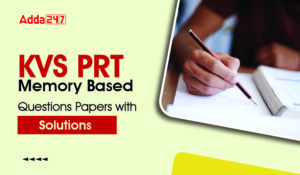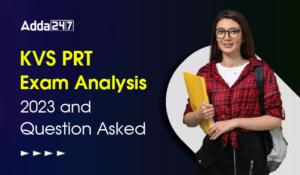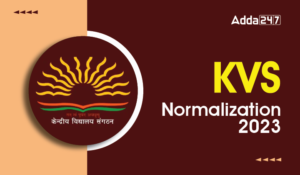Latest Teaching jobs   »   Maths Questions for All Teaching Exam...

# Maths Questions for All Teaching Exam :14th February 2019

Dear Students!!! There is most general as well as a scoring section in all the competitive entrance examinations in the teaching field i.e “Mathematics”.Because in this section only one thing is work i.e your accuracy and that could be nourished with the daily practice. So, for this, we are providing you the daily quiz for all teaching exams i.e CTET Exam 2019, DSSSB ,KVS,STET Exam.

GET 5% DISCOUNT on CTET Prime :
Use This Code TEACH5
Q1. By melting a solid lead sphere of diameter 12 cm, three small spheres are made whose diameters are in the ratio 3 : 4 : 5. The radius (in cm) of the smallest sphere is
(a) 3
(b) 6
(c) 1.5
(d) 4
Q2. The ratio of the surface area of a sphere and the curved surface area of the cylinder circumscribing the sphere is
(a) 1 : 2
(b) 1 : 1
(c) 2 : 1
(d) 2 : 3
Q3. The radii of the base of two cylinders A and B are in the ratio 3 : 2 and their height in the ratio x : 1. If the volume of cylinder A is 3 times that of cylinder B, the value of x isQ4. The radius of base and slant height of a cone are in the ratio 4 : 7. If slant height is 14 cm then the radius (in cm) of its base is
(a) 8
(b) 12
(c) 14
(d) 16
Q5. The ratio of the volume of a cube and of a solid sphere is 363 : 49. The ratio of an edge of the cube and the radius of the sphere is
(a) 7 : 11
(b) 22 : 7
(c) 11 : 7
(d) 7 : 22
Q6. ABC is a triangular field and D, E, F are the mid-points of the sides BC, CA, AB respectively. The ratio of the areas of ABC and DEF is :
(a) 4 : 1
(b) 5 : 1
(c) 3 : 1
(d) can’t be determined
Q7.The length and breadth of a rectangular field are 120 m and 80 m respectively. Inside the field, a park of 12 m width is made around the field. The area of the park is :Q8. The circumference of the front wheel of a cart is 30 ft long and that of the back wheel is 36 ft long. What is the distance travelled by the cart, when the front wheel has done five more revolutions than the rear wheel?
(a) 20 ft
(b) 25 ft
(c) 750 ft
(d) 900 ft
Q9. The figure below has been obtained by folding a rectangle. The total area of the figure (as visible) is 144 square meters. Had the rectangle not been folded, the current overlapping part would have been a square. What would have been the total area of the original unfolded rectangle?(a) 128 square meters
(b) 154 square meters
(c) 162 square meters
(d) 172 square meters
Q10. A circular road is constructed outside a square field. The perimeter of the square field is 200 ft. If the width of the road is 7√2 ft. and cost of construction is Rs. 100 per sq. ft. Find the lowest possible cost to construct 50% of the total road.
(a) Rs.70,400
(b) Rs.1,25,400
(c) Rs.1,40,800
(d) Rs.2,35,400

Sharing is caring!

Thank You, Your details have been submitted we will get back to you.

### TOPICS:

•KVS PRT Memory Based Questions Papers wi...
•KVS PRT Exam Analysis 2023 & Asked Q...
•KVS Normalization Process 2023, New Guid...
•KVS PRT Exam Analysis 2023 & Asked Q...
•KVS PRT Exam Analysis 2023 & Asked Q...
•KVS PRT Exam Analysis 2023 & Asked Q...